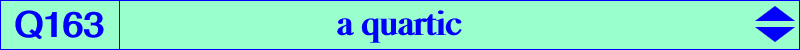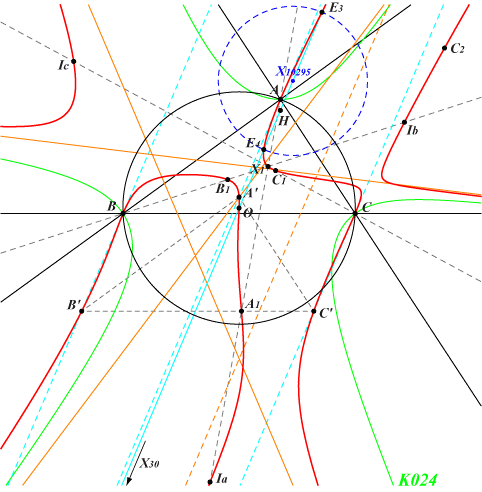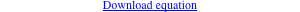too complicated to be written here. Click on the link to download a text file.X(1), X(3), X(30), X(40894), X(40895) excenters infinite points of K024 Ua, Ub, Uc as in Table 18 other points belowLet P be a point with circumcevian triangle PaPbPc. Denote by Ea, Eb, Ec the centers X(30) of triangles PaBC, PbCA, PcAB respectively. PaPbPc and EaEbEc are perspective if and only if P lies on the quartic Q163 (together with the circumcircle). In other words, the parallels at Pa, Pb, Pc to the Euler lines of triangles PaBC, PbCA, PcAB concur. Q163 has four real asymptotes. One is parallel to the Euler line of ABC and three are parallel to those of K024 hence they are parallel to the sidelines of the Morley triangle. Other points on Q163 : • A', B', C' : images of A, B, C under the translation that sends H to O. • A1, B1, C1 and A2, B2, C2 : intersections of the sidelines of A'B'C' with the internal and external bisectors of ABC respectively. • E3, E4 on the Euler line. The circle with diameter E3E4 has center X(10295) and is orthogonal to the circumcircle. Hence, E3 and E4 are inverse in the circumcircle. These points are now X(40895), X(40894) in ETC (2021-01-03). They also lie on the circum-hyperbola passing through X(i) for i in {250, 841, 3431, 18850}. See the related quartics Q162, Q164 and Q165. See Q023 for a generalization.